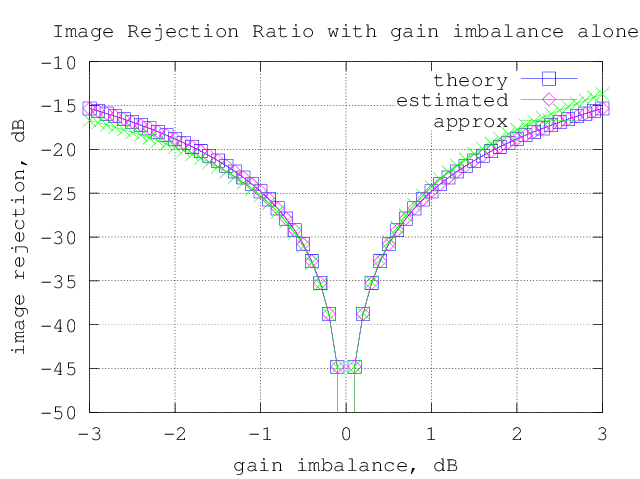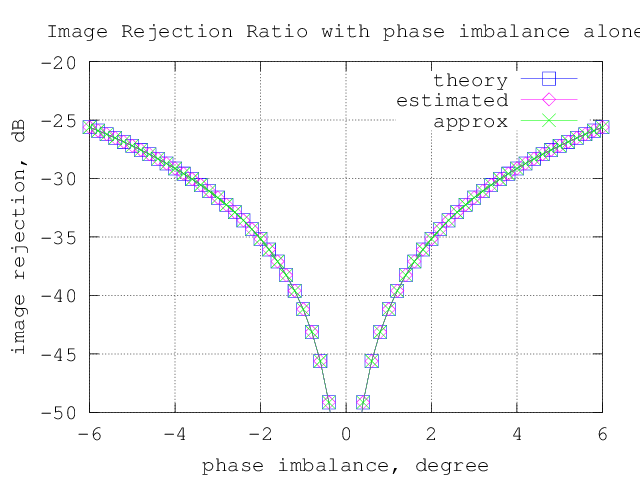(2 votes, average: 5.00 out of 5)Loading ...

# Image Rejection Ratio (IMRR) with transmit IQ gain/phase imbalance

by on January 31, 2013

The post on IQ imbalance in transmitter, briefly discussed the effect of amplitude and phase imbalance and also showed that IQ imbalance results in spectrum at the image frequency. In this article, we will quantify the power of the image with respect to the desired tone (also known as IMage Rejection Ratio IMRR) for different values of gain and phase imbalance.

## System Model

Consider an IQ modulator having gain of  and on each arm and phase imbalance of  as shown in figure below.Figure : IQ modulator with gain and phase imbalance

The output signal is,

.

Considering an ideal IQ demodulator multiplying the received signal  with and respectively,

.

.

Ignoring the common term  and writing the base band equivalent form,

.

This is the model for transmit IQ imbalance.

## Image Rejection Ratio (IMRR) with transmit IQ imbalance

By sending a complex sinusoidal , and by taking ratio of the power of the signal at the image frequency   and desired frequency  , the image rejection ratio can be computed.

Let and correspondingly,  and .

Finding the  component

To find the  component, multiply the received signal  with  and integrate over period .

The power of the  component is,

Finding the  component

To find the  component, multiply the received signal  with  and integrate over period .

The Image Rejection Ratio (IMRR) is

.

Substituting  and  with variable  and , the equation simplifies to,

.

A useful approximation to IMRR

When there is no phase imbalance i.e , the equation reduces to,

.

When there is no gain imbalance i.e , the equation reduces to,

.

As these two are independent, they can be added to give an approximate value of Image Rejection Ratio.

Summarizing, the Image Rejection Ratio for a given value of gain imbalance  and phase imbalance is,

.

Simulation Results

Simple Matlab/Octave code plotting the simulated and theoretical values of Image Rejection for different values of gain and phase imbalance.

clear; close all

N  = 64;
fm = 2;
phiDeg_v  = [-6:.2:6];
[tt phiDeg_zeroIdx  ] = min(abs((phiDeg_v-0)));

for (jj = 1:length(phiDeg_v))
phiDeg     = phiDeg_v(jj);
epsilonLin = gammaLin -1 ;

% transmitted signal
xt        = exp(j*2*pi*fm*[0:N-1]/N);
% received signal with IQ imbalance
xht       = xht_re + j*xht_im;

% taking ifft() to find the +fm and -fm components
yF        = fft(xht,N);
y_pfm     = yF(fm+1);
y_nfm     = yF(N-fm+1);

est_imrr_lin    = (abs(y_nfm)./abs(y_pfm))^2;

est_imrr_dB(ii,jj)    = 10*log10(est_imrr_lin);
theory_imrr_dB(ii,jj) = 10*log10(theory_imrr_lin);
approx_imrr_dB(ii,jj) = 10*log10(approx_imrr_lin);
end
end

figure
xlabel('gain imbalance, dB'); ylabel('image rejection, dB'); grid on;
legend('theory','estimated','approx')
title('Image Rejection Ratio with gain imbalance alone');
axis([-3 3 -50 -10]);

figure
xlabel('phase imbalance, degree'); ylabel('image rejection, dB'); grid on;
legend('theory','estimated','approx')
title('Image Rejection Ratio with phase imbalance alone');
axis([-6 6 -50 -20]);Figure : Image Rejection Ratio (IMRR) with gain imbalance aloneFigure : Image Rejection Ratio (IMRR) with phase imbalance alone

Observations

1) The approximate expression holds good for reasonable values of gain and phase imbalance.

2) As a rule of thumb, the following numbers are useful :

- For 1 degree of phase imbalance, the Image Rejection Ratio (IMRR) is around -41dB

- For 1dB of gain imbalance, the Image Rejection Ratio (IMRR) is around -25dB

## References

 Cavers, J.K.; Liao, M.W.; , “Adaptive compensation for imbalance and offset losses in direct conversion transceivers,” Vehicular Technology, IEEE Transactions on , vol.42, no.4, pp.581-588, Nov 1993 doi: 10.1109/25.260752

 Table of trignometric identities http://www.sosmath.com/trig/Trig5/trig5/trig5.html

D id you like this article? Make sure that you do not miss a new article by subscribing to RSS feed OR subscribing to e-mail newsletter. Note: Subscribing via e-mail entitles you to download the free e-Book on BER of BPSK/QPSK/16QAM/16PSK in AWGN.